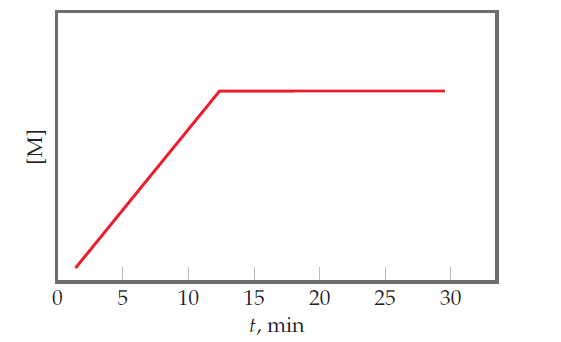×
Get Full Access to Chemistry: The Central Science - 12 Edition - Chapter 14 - Problem 4e
Get Full Access to Chemistry: The Central Science - 12 Edition - Chapter 14 - Problem 4e

×

# You perform the reaction monitor the production of M overISBN: 9780321696724 27

## Solution for problem 4E Chapter 14

Chemistry: The Central Science | 12th Edition

• Textbook Solutions
• 2901 Step-by-step solutions solved by professors and subject experts
• Get 24/7 help from StudySoup virtual teaching assistantsChemistry: The Central Science | 12th Edition

4 5 1 431 Reviews
11
2
Problem 4E

You perform the reaction K + L → M, monitor the production of M over time, and then plot this graph from your data:Is the reaction occurring at a constant rate from t = 0 to t = 15 min? Explain. (b) Is the reaction completed at t = 15 min? Explain.

Step-by-Step Solution:
Step 1 of 3

PSYCH STATISTICS CHAPTER ONE Populations and Samples ● statistics = mathematical procedures for organizing, summarizing, and interpreting info ● population = set of all the individuals of interest in a study ○ can be pretty big ● sample = set of people selected from a population, usually intended to represent the population ● variable = a characteristic or condition that changes or has different values for different individuals ○ ex. height, weight, gender, personality ● data = measurements or observations ● data set = collection of measurements or observations ● datum = single measurement of observation ○ often called a score

Step 2 of 3

Step 3 of 3

##### ISBN: 9780321696724

The answer to “?You perform the reaction K + L ? M, monitor the production of M over time, and then plot this graph from your data: 1. Is the reaction occurring at a constant rate from t = 0 to t = 15 min? Explain. 2. (b) Is the reaction completed at t = 15 min? Explain.” is broken down into a number of easy to follow steps, and 55 words. Chemistry: The Central Science was written by and is associated to the ISBN: 9780321696724. This full solution covers the following key subjects: data, graph, monitor, perform, plot. This expansive textbook survival guide covers 49 chapters, and 5471 solutions. This textbook survival guide was created for the textbook: Chemistry: The Central Science, edition: 12. The full step-by-step solution to problem: 4E from chapter: 14 was answered by , our top Chemistry solution expert on 04/03/17, 07:58AM. Since the solution to 4E from 14 chapter was answered, more than 304 students have viewed the full step-by-step answer.

Unlock Textbook Solution